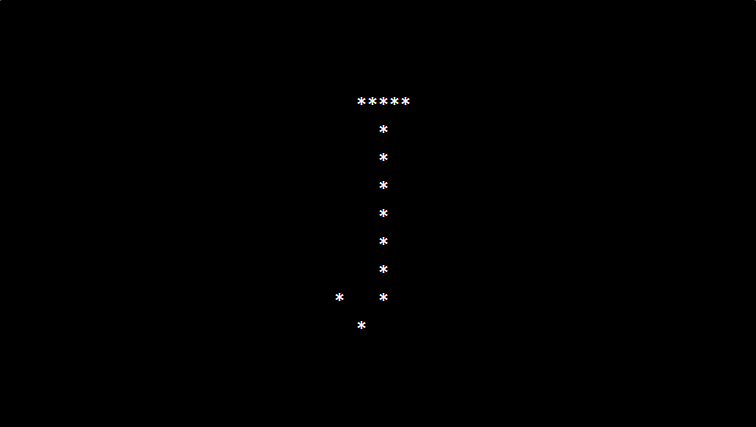# PHP program to print alphabet pattern J

In this article write a PHP program to print alphabet pattern J. This Program first takes the numbers of rows and then prints pattern using nested for loops.

## PHP program to print alphabet pattern J

```<?php
echo "<pre>";
for (\$row = 0; \$row < 9; \$row++) {
for (\$col = 0; \$col <= 9; \$col++) {
if ((\$col == 5 AND \$row != 8) OR (\$row == 0 AND \$col > 2 AND \$col < 8) OR (\$row == 7 AND \$col == 1) OR (\$row == 8 AND \$col == 3)) {
echo "*";
} else {
echo "&nbsp;";
}
}
echo "<br/>";
}
echo "</pre>";
?>
```

### Output: